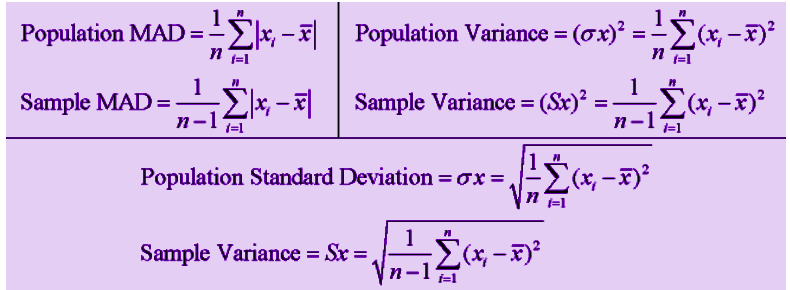# Population and Sample

In statistics as well as in quantitative methodology, the set of data are collected and selected from a statistical population with the help of some defined procedures. There are two different types of data sets namely, population and sample. So basically when we calculate the mean deviation, variance and standard deviation, it is necessary for us to know if we are referring to the entire population or to only sample data. Suppose the size of the population is denoted by ‘n’ then the sample size of that population is denoted by n -1. Let us take a look of population data sets and sample data sets in detail.

## Population

It includes all the elements from the data set and measurable characteristics of the population such as mean and standard deviation are known as a parameter. For example, All people living in India indicates the population of India.

There are different types of population. They are:

• Finite Population
• Infinite Population
• Existent Population
• Hypothetical Population

Let us discuss all the types one by one.

### Finite Population

The finite population is also known as a countable population in which the population can be counted. In other words, it is defined as the population of all the individuals or objects that are finite. For statistical analysis, the finite population is more advantageous than the infinite population. Examples of finite populations are employees of a company, potential consumer in a market.

### Infinite Population

The infinite population is also known as an uncountable population in which the counting of units in the population is not possible. Example of an infinite population is the number of germs in the patient’s body is uncountable.

### Existent Population

The existing population is defined as the population of concrete individuals. In other words, the population whose unit is available in solid form is known as existent population. Examples are books, students etc.

### Hypothetical Population

The population in which whose unit is not available in solid form is known as the hypothetical population. A population consists of sets of observations, objects etc that are all something in common. In some situations, the populations are only hypothetical. Examples are an outcome of rolling the dice, the outcome of tossing a coin.

 Related Links Statistics Mean Probability Probability And Statistics

## Sample

It includes one or more observations that are drawn from the population and the measurable characteristic of a sample is a statistic. Sampling is the process of selecting the sample from the population. For example, some people living in India is the sample of the population.

Basically, there are two types of sampling. They are:

• Probability sampling
• Non-probability sampling

### Probability Sampling

In probability sampling, the population units cannot be selected at the discretion of the researcher. This can be dealt with following certain procedures which will ensure that every unit of the population consists of one fixed probability being included in the sample. Such a method is also called random sampling. Some of the techniques used for probability sampling are:

• Simple random sampling
• Cluster sampling
• Stratified Sampling
• Disproportionate sampling
• Proportionate sampling
• Optimum allocation stratified sampling
• Multi-stage sampling

### Non Probability Sampling

In non-probability sampling, the population units can be selected at the discretion of the researcher. Those samples will use the human judgements for selecting units and has no theoretical basis for estimating the characteristics of the population. Some of the techniques used for non-probability sampling are

• Quota sampling
• Judgement sampling
• Purposive sampling

### Population and Sample Examples

• All the people who have the ID proofs is the population and a group of people who only have voter id with them is the sample.
• All the students in the class are population whereas the top 10 students in the class are the sample.
• All the members of the parliament is population and the female candidates present there is the sample.

### Population and Sample Formulas

We will demonstrate here the formulas for mean absolute deviation (MAD), variance and standard deviation based on population and given sample. Suppose  n denotes the size of the population and n-1 denotes the sample size, then the formulas for mean absolute deviation, variance and standard deviation are given by;### Difference between Population and Sample

Some of the key differences between population and sample are clearly given below:

 Comparison Population Sample Meaning Collection of all the units or elements that possess common characteristics A subgroup of the members of the population Includes Each and every element of a group Only includes a handful of units of population Characteristics Parameter Statistic Data Collection Complete enumeration or census Sampling or sample survey Focus on Identification of the characteristics Making inferences about the population

For more information on statistics, register with BYJU’S – The Learning App and also watch some interesting videos to learn with ease.